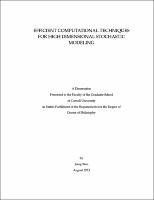## Efficient Computational Techniques For High Dimensional Stochastic Modeling##### Abstract
Modeling of physical systems in the presence of uncertainties is critical in many respects. Therefore it is necessary to quantitatively characterize these uncertainties. There are two major types of problems with respect to uncertainty quantification: inverse problems and forward problems. In inverse problems, it is essential to estimate the uncertainties arising from limited observation data. In forward problems, the main objective is to understand how input uncertainties propagate and how they affect model responses. In spite of tremendous progress made in the past few decades, the problems arising from highdimensional input remain a long-standing challenge. The focus of this thesis is developing an efficient computational framework to overcome the curse of dimensionality in both inverse and forward problems. For inverse problems with high-dimensional input, we develop a Bayesian computational framework in which the input field is discretized using a sparse grid and represented by local basis functions associated with the collocation points. Based on the hierarchical property of sparse grids, a sequence of hierarchical Bayesian models from coarse to fine scales is proposed. The sparse grid also provides an efficient way of finding an optimal choice of basis functions to approximate the spatially varying input, which leads to an adaptive refinement strategy. As a result, it reduces the dimensionality of the inverse problem and the computational cost of Bayesian inference. This Bayesian computational approach is nonparametric and thus is applicable to various spatially varying parameter estimation problems. For forward problems with high-dimensional input, probabilistic graphical models, which have been extensively used in machine learning and information science, are employed to approximate the high-dimensional joint probability density functions that exist in uncertainty quantification. We combine the graphical models and a popular model reduction technique, KarhunenLo` ve expansion, to construct accurate stochastic input model for non-Gaussian e random fields. Furthermore, we develop a probabilistic graphical model based methodology for uncertainty quantification in the presence of both highdimensional stochastic input and multiple scales. In this framework, the stochastic input and model responses are treated as random variables. Their relationships are modeled by graphical models which give explicit factorization of the high-dimensional joint probability distribution. In this way, an efficient inference algorithm, belief propagation, is applied to infer the statistics of model responses directly on the graph without involving sampling-based methods and expensive deterministic solvers.
2013-08-19
##### Committee Chair
Zabaras, Nicholas John
##### Committee Member
Earls, Christopher J
Warner, Derek H.
##### Degree Discipline
Mechanical Engineering
##### Degree Name
Ph. D., Mechanical Engineering
##### Degree Level
Doctor of Philosophy
##### Types
dissertation or thesis